Next: Comments Up: Exploring the Basics Previous: Initiating a Darwin Session

# Basic Terminology and Arithmetic Operators

We start by issuing commands to Darwin which compute simple arithmetic operations. The basic data objects in all programs are symbols and constants. A constant in Darwin is any number. For example,
1.1, 5, -999, 88382932
A symbol is a letter (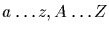) or an underscore symbol (_). For example,
a, b, m, M, Z, _
An operator specifies what should be done to a set of constants and symbols. The following are all operators in Darwin,
+, -, *, /, =, <, >, <>, <=, >=
Together, symbols and constants written in the correct syntax form expressions. If you type the simple expression,

> 1+1

followed by the return key1.1, Darwin responds by supplying a fresh prompt on the line below and then waits for more instructions. Either the user must continue extending this expression or they must enter a semicolon followed by the return key.
> ;
2

The expression 1+1 combined with the semicolon (;) is our first example of a statement in Darwin. All Darwin statements are built from commands, expressions and either a terminating semicolon (<tex2htmlverbmark>4<tex2htmlverbmark>) or colon (<tex2htmlverbmark>5<tex2htmlverbmark>). When Darwin receives the statement, it evaluates the expression and echos the result to the terminal. If we had instead choosen to terminate our expression with a colon, Darwin would evaluate the expression but suppresses the (surprising) answer.
> 1 + 1:

The utility of the colon will be made clearer in later sections when we begin to write routines and loops. The colon allows us to control which statements are echoed to the screen and which are executed silently.

There are a wide range of operators we can use to form expressions in Darwin. Tablelists some of the more commonly used ones.

> 1 * 2 * 3 * 4;
24
> 4!;
24
> 2^5 + 2^6;
96
> round(4.9999) * round(4.9999);
25


Table: Some arithmetic operations offered by Darwin.
1.1
 Operation Symbol Example Result addition + 101+27 128 subtraction - 15-3 12 multiplication * 5*3 15 division / 6/2 3 exponentiation ^ or ** 278 256 natural exponentiationexp(3) 20.0855 absolute value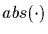abs(-6.7) 6.7 factorial ! 5! 120 square root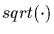sqrt(16) 4 natural logarithm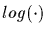or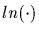log(1) 0 logarithm base 10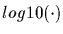log10(100) 2 rounding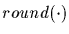round(4.99999) 5

Any number of return or space keystrokes may be entered by the user before a semicolon (or colon) is entered. These white space characters, as they are called, do not affect the calculation in any way. For example,

> 8 +3
>        -5
>      *   8 /      2
>
>    ;
-9

produces the same result as
> 8+3-5*8/2;
-9

With most computers, there is an overall limit on the length of a single line. This is typically is on the order of two or three hundred keystrokes. Entering a statement which exceeds this limit does not pose a problem since the semicolon allows us to split the entry over several lines.
> 1 + 2 + 3 + 4 + 5 + 6 + 7 + 8 + 9 + 10 + 11 + 12 + 13 +
> 14 + 15 + 16 + 17 + 18 + 19 + 20 + 21 + 22 + 23 + 24 +
> 25 + 26 + 27 + 28 + 29 + 30 + 31 + 32 + 33 + 34 + 35 +
> 36 + 37 + 38 + 39 + 40 + 41 + 42 + 43 + 44 + 45 + 46 +
> 47 + 48 + 49 + 50;
1275

Of course, in this case the true mathematicians amongst us would have opted for the following more elegant and fewer keystroke solution anyway.
> (50*(50+1))/2;
1275

Beware, it is easy to make errors when splitting entries over several lines. If you enter
> 8 + 3
> 2 + 5;
syntax error:
2 + 5;
^

then you will have officially experienced your first syntax error in Darwin since there is neither a semicolon, colon nor operator between the 3 and the 2. When any such error occurs, Darwin responds with a brief message consisting of your input and a caret symbol (^) at the point in your statement where it first became confused. It then gives you a fresh prompt and ignores your previous entry.

When performing a sequence of statements, making use of the double quote symbol (<tex2htmlverbmark>9<tex2htmlverbmark>) will sometimes save you keystrokes. A single double quote symbol refers to the result of the last statement. Two double quote symbols (<tex2htmlverbmark>10<tex2htmlverbmark>) refer to the result of the second last statement and the result preceding this is referred to by (<tex2htmlverbmark>11<tex2htmlverbmark>). The example below shows how these can be used to generate the elements of the famous Fibonacci sequence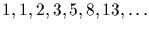.

> 1;
1
> 1;
1
> " + "";
2
> " + "";
3
> " + "";
5
> " + "";
8


When submitting longer arithmetic expressions, users must remember to respect the order of operations. For instance, the expression

> 5 + 8 * 3 / 2;

does not evaluate to 19.5 but to 17. This is because multiplication and division have higher precedence than addition and subtraction. If we first wanted the addition of 5 and 8performed before the multiplication and division, we would rewrite the expression using parenthesis as
> 5 + 8 * 3 / 2;
17
> (5 + 8) * 3 / 2;
19.5000

Tableshows the order of operations in Darwin.

Table: Order of operations in Darwin listed from highest to lowest precedence.
1.1
 Operation Symbol parenthesis (,) exponentiation ^ or ** negation (unary subtraction) - multiplication/division *,/ addition/subtraction +,-

Subtle errors can be caused by forgotten parentheses. Beware of the following pitfalls:

> -5 ^ 2;             # Negation has a lower precedence than exponentiation
-25
> (-5) ^ 2;           # The parenthesises force the exponentiation to use -5 instead
25
> -5 ** 2;            # Same thing, but using the other exponentiation operator.
-25
> -5 * -2;            # Negative numbers must be parenthesized when following
syntax error:
-5 * -2;
^
> -5 * (-2);          #    an operator.
10
> 2 ^ 2 ^ 2;          # Towers of exponents must be parenthesized.
syntax error:
2 ^ 2 ^ 2;
^
> 2 ^ ( 2 ^ 2 );
16


A complete list of all the mathematical functions built into Darwin is located in the reference guide under the section §Mathematical Functions in Part- The Reference Guide.Next: Comments Up: Exploring the Basics Previous: Initiating a Darwin Session
Gaston Gonnet
1998-09-15